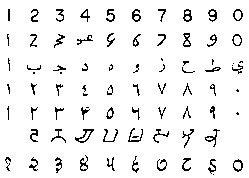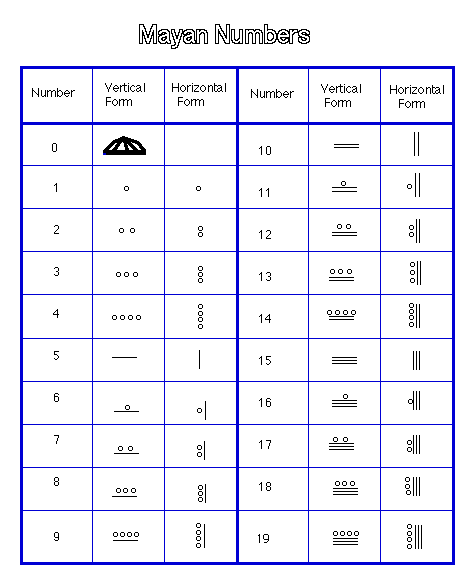## by Martin Flashman

I. What do we understand by the number 7?

1. Distinguish the number from the numeral:
1. Symbols , words, and other designations.

2. Examples of numerals for the number seven.
• Various Arabic numerals

•• Roman Numerals I, II, III, IV, V, VI, VII
• Egyptian numerals

•• Mayan Numerals

•• Binary Notation: 1,10,11,100,101,110,111
• Different Languages: ....

3. What defines the number 7? Webster's definitions.

4. Encyclopedia Britannica .

2. Meeting the number 7 in some common places.
1. On a calendar.
2. On a color wheel.
3. In games and sports.
• 7 fielders in baseball (not part of the "battery").
• 7 players in indoor soccer
• 7 line players in football
• 7 card poker games (7 card stud and Texas Hold'em)
4. In mystical/religious writings
• lucky 7
5. In music and dance:
• The seven notes of a diatonic scale.
• The dance of the seven veils.
6. In literature:
• The House of Seven Gables
• Seven Samurai
• Seven Brides for Seven Brothers
• Snow White and the Seven Dwarfs
7. In geography:
• The seven seas.
• The seven (?) continents.
• The seven wonders of the world.
8. In committee  organization:

9. The HSU Math department has 7 standing committees.
Personnel

Kieval Lecture                                Scholarship/prizes

Curriculum

Technology                          Statistics                Teacher Preparation

3. Definitions of the number 7.
1. By itself.
• A cardinal number of a set.
• An ordinal number from an ordered set.
2. By its  unique properties:

3. The unique number such that .....
4. By recognizing its qualities in a variety of contexts.
• Common
• Mathematical
II. Some common contexts for finding the number 7.
1. Musical structures.

2. An atonal chord structure.
3. Committee structures.

4. A committee structure.
5. Scheduling structures.

6. A design for scheduling classes?
7. Color Wheel/Triangle. (show with GSP)

8.
III. Some Mathematical Contexts for  finding the number 7.
1. A Geometric structure
• A graphical geometry.
• Visualizing this geometry. (GSP)
2. A Geometric structure
• A triangular geometry.
• Visualizing this geometry. (GSP)
3. Some projective geometric ideas based on a focus.
• A line through the focus appears as a point.
• A plane through the focus appears as a line.
• Two points determine a plane including the focus.
• Any two planes through the focus determine a line through the focus.
• Definitions:

• A line through the focus is called a projective point (a P-point) and
A plane through the focus is called a projective line (a P-line).

A projective plane is the geometric object made up of the collection of P-points and P-lines.

• In a projective plane,

• any two P-points determine a unique P-line and any two P-lines determine a unique P-point.
4. Some algebraic descriptions of lines and planes in 3 dimensions.
• A plane through the origin has an equation of the form Ax + By + Cz  = 0, where [A,B,C] is not [0,0,0]. The triple [uA,uB,uC] will determine the same plane as long as u is not 0.

• For example, [1,0,1] determines the plane with equation X + Z = 0.
• A line through the origin has the equation of the form (X,Y,Z) = (a,b,c) t where (a,b,c) is not (0,0,0).The triple (ua,ub,uc)will determine the same line as long as u is not 0.

• For example, (1,0,-1) deteremines the line with equation (X,Y,Z)=(1,0,-1)t.
• A P-line has an equation of the form Ax + By + Cz  = 0, where [A,B,C] is not [0,0,0].

• The triple [uA,uB,uC] will determine the same plane as long as u is not 0.

We'll call [A,B,C] homogeneous coordinates of the P-line.

For example, [1,0,1] are homogeneous coordinates for the P-line determined by the plane with equation X + Z = 0.

• A P-point has the equation (X,Y,Z) = (a,b,c) t where (a,b,c) is not (0,0,0).The triple (ua,ub,uc) will determine the same line as long as u is not 0.

• We'll call <a,b,c> homogeneous coordinates of the P- point.

For example, <1,0,-1> are homogeneous coordinates for the P-point determined by the line with equation (X,Y,Z) = (1,0,-1) t.

• A P-point lies on a P line or a P -line passes through the the P-point if and only if  Aa+Bb+Cc= 0 where [A,B,C] are homogeneous coordinates for the P-line and <a,b,c> are homogeneous coordinates for the P-point.

• For example, the P-point <1,0,-1> lies on the P-line [1,0,1].
• NOTE: All of the discussion works as long as the symbols A,B,C, a,b, and c represent elements of a field, that is, a set with two operations that work like the real or rational numbers in terms of addition and multiplication.
5. A field with two elements:   F2 = {0,1}.

6.
 + 0 1 x 0 1 0 0 1 0 0 0 1 1 0 1 0 1

A projective plane using F2 has  exactly 7 points:

<0,0,1>, <0,1,0>, <0,1,1>,<1,0,0>,<1,0,1>,<1,1,0>,<1,1,1>.

A projective plane using F2 has exactly 7 lines:

[0,0,1], [0,1,0], [0,1,1], [1,0,0], [1,0,1], [1,1,0], [1,1,1].

This projective plane satisfies the geometric structure properties.

 Lines\Points <0,0,1> <0,1,0> <0,1,1> <1,0,0> <1,0,1> <1,1,0> <1,1,1> [0,0,1] X X X [0,1,0] X X X [0,1,1] X X X [1,0,0] X X X [1,0,1] X X X [1,1,0] X X X [1,1,1] X X X

We can visualize the projective plane  using F2 using 7 points of the unit cube in ordinary 3 dimensional coordinate geometry. (GSP)

THE END.